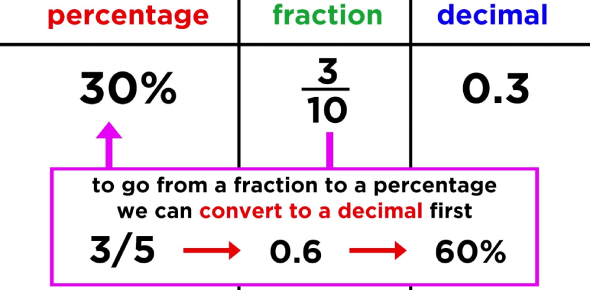# Fractions, Decimals, Percentages

8 Questions | Total Attempts: 6332Settings.

• 1.
What is 2/3 of 12?
• A.

4

• B.

6

• C.

8

• D.

7

• E.

12

• 2.
What is 50% as a fraction?
• A.

1/2

• B.

1/3

• C.

2/10

• D.

5/100

• E.

1/10

• 3.
What is 1/3 as a decimal?
• A.

0.5

• B.

1.3

• C.

0.1

• D.

1.3

• E.

0.33

• 4.
What is the same as 2/5?
• A.

4/10

• B.

12/15

• C.

1/10

• D.

5/2

• E.

3/8

• 5.
Mathew had seven pages of his book left to read.  There were 100 pages in total.  What percentage of pages did he have left?
• A.

70%

• B.

1.7%

• C.

77%

• D.

100%

• E.

7%

• 6.
If I have 45 minutes of lunchtime left to play ball tag with Riccardo, what fraction of my lunch break does that leave us?
• A.

45/60

• B.

2/5

• C.

3/4

• D.

5/10

• E.

2/3

• 7.
What is 3/10 as a decimal?
• A.

0.1

• B.

1.3

• C.

3.1

• D.

3.0

• E.

0.3

• 8.
Write a short explanation of how to convert fractions to decimals.
Related TopicsBack to top